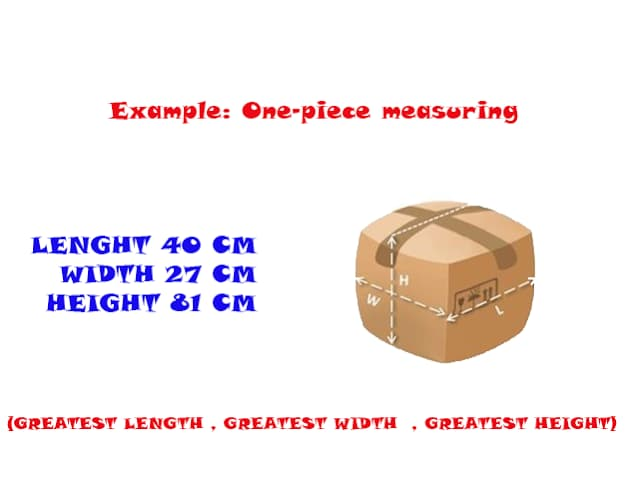# Calculating chargeable weight

You may not have any idea about the thing called chargeable weight. Calculating the chargeable weight of air cargo shipment is a basic air cargo shipping skill. Airline or air cargo agents who accept shipment from a shipper use this calculation process by comparing the gross weight of a shipment and the volume weight of the same shipment, the higher one is used and called chargeable weight.

In this article, I am going to explain to you air freight chargeable weight step by step calculating. Before I start explaining that, you may need to understand some basic air cargo rating principles.

Basic Air Cargo Tariff Definitions Related to Chargeable Weight

1- Gross Weight ( High-Density Cargo)

The gross weight is used as the chargeable weight in the case of cargo which is heavy in relation to its volume such as gold, machinery, metal parts etc. This type of cargo is called High-Density Cargo.

HIGH-DENSITY CARGO is cargo which weighsEqual Or More Than 166.666 kgs per m3

or

2- Volume Weight (Low-Density Cargo)

In the case of cargo which is bulky in relation to its weight, such as hats, woolen pullovers, etc, the chargeable weight will be established on the basis of volume rather than gross weight.

The VOLUME WEIGHT will be calculated and used as the chargeable weight. This type of cargo is calledLOW-DENSITY CARGO.

LOW-DENSITY CARGO is cargo which weighs LESS THAN166.666 kgs per m3

or

3-Chargeable Weight

The chargeable weight is the actual gross weight or volume weight, whichever is higher

The MINIMUM WEIGHT is a rate published together with certain rate categories in The Air Cargo Tariff Online solutions by IATA . or can be published by airline for customers at its air cargo sales points.

Rounding of Chargeable Weights

The chargeable weight must be rounded up as follows:

Examples

Volume/ Gross Weight 100.2 kgs= Chargeable Weight 100.5 kgs

Volume/Gross weight 100 lbs 2 ounces =Chargeable Weight 101 lbs

Note: A calculated volume weight of 0.501 kgs,0.001 kgs , or 0.001 lb must be rounded up to the next higher full, half kg or higher full pound respectively

Examples

A Volume weight of 315.001 kgsmust be rounded upto 315.5 kgs.

A volume weight of 1670.001 lbsmust be rounded up to 1671 lbs

Calculating the Chargeable Weight

The following calculations must be made to determine the volume weight of one or several pieces of cargo:

A- Consignments of One PieceSTEP 1-   Measure the piece along with its

(GREATEST LENGTH, GREATEST WIDTH, GREATEST HEIGHT)

Regardless of its geometric shape.

STEP 2 - Round off dimensions as follows:

Example: One-piece measuring

40.3 cm x 26.5 cm x 80.6 cm

This figure should be rounded like this :

= 40 cm x 27 cm x 81 cm.

STEP 3

Multiply rounded-off measurements to obtain the volume of the piece.

Examples.

= 40 cm x 27 cm x 81 cm = 87,480 Cu Cm

STEP 4

The limit between high- and low-density cargo being: 6000 Cu Cm per kg or 366 Cu In per kg or 166 Cu In per Lb.

The volume weight is established by dividing the volume of the piece by the appropriate factor, ie.

Why dividing by 6000

Because one  1 kilogram = 6000 Cubic Centimeters  (Volume Weight)

Important

The volume weight shall be calculated up to three decimal places before rounding off ( see Red numbers down).

Example.

Volume = 87,480 Cu Cm

87,480 Cu Cm Divided by 6000 =14.580 kgs

STEP 5. Round off volume weight in the same manner as gross weight

Example

14.580 kgs = 15 kgs

Let us imagine that the package gross weight was 10 kgs, and the package volume weight as we calculated before is 15 kgs. For sure the chargeable weight will be the higher one which is 15 kgs.

Are you ready to start calculating chargeable weight for two pieces?

Let's go.

B. Consignments of Two or More Pieces

A consignment may consist of several pieces representing both high- and low-density cargo, if the entire consignment is to be charged at the same rate, Then the chargeable weight will be the total gross weight or the total volume weight, whichever is higher.

Example

2 packages sent as one consignment:

 Package A Package B GROSS Weight 30 kgs 40 kgs Dimension 90 cm × 50 cm× 70 cm Diameter 50cm ×Height  80 CM

Note : Drum diameter is considered as a width and length

STEP 1

Establish the total volume of the entire consignment and round it off if necessary

Package

90 cm x 50 cm x 70 cm = 315,000 cu cm

Package B

50 cm x 50 cm x 80 cm = 200,000 cu cm

Package A Volume Weight + Package B Volume Weight

= 515000 cu cm

Step 2 Establish the total volume weight of the entire consignment :

Now

515000 cu cm divided by 6000 = 85.833 kgs

Let us a roundup it  = 86 kgs.

In this example the total volume weight = 86 kgs, it is higher than the total gross weight ( which is 70 kgs) and will be therefore chargeable weight for this shipment.

 For 2 pieces Total Gross Weight 70kgs. Total Volume Weight 86kgs. Chargeable Weight 86kgs.

 The chargeable weight must always be shown on the air way bill.